As we all know, Vlookup formula is used to lookup the value from the left most column. In advanced Vlookup function, we combine other functions for the improved outcome.

In this article, you will learn how you can use Vlookup to solve the problem in the advance way. This may even help you to create a dynamic dashboard.

Below is the image of Advance Vlookup (refer to Image 1).

In this Example, we have created helper column, defined the range name and on the basis of two lookup value we are getting the result.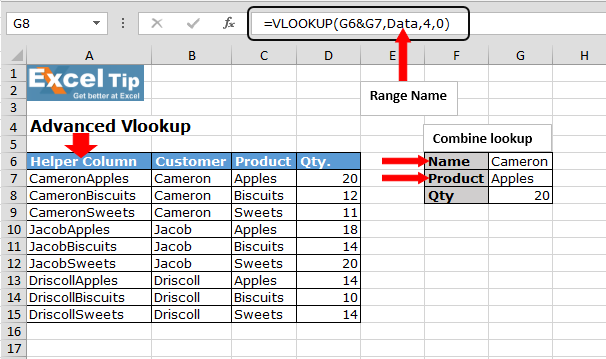Let’s understand from the start:-

We have data in which we have Customer name, product and quantity in the range A6:C15. In the other side, we have two drop down list: - first is customer name and second is product, so we want to retrieve the quantity as per the selection.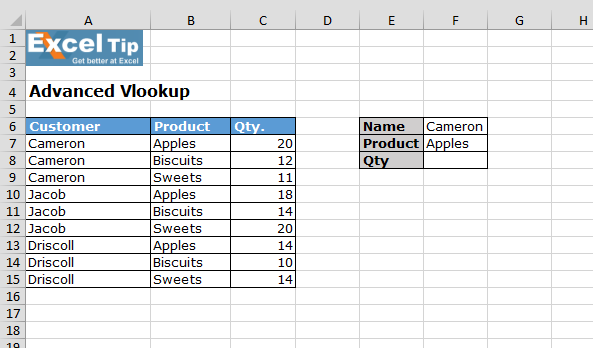Step 1:Insert helper column,

• Insert a column before A column
• Write the header name: Helper Column
• Enter the formula: =B7&C7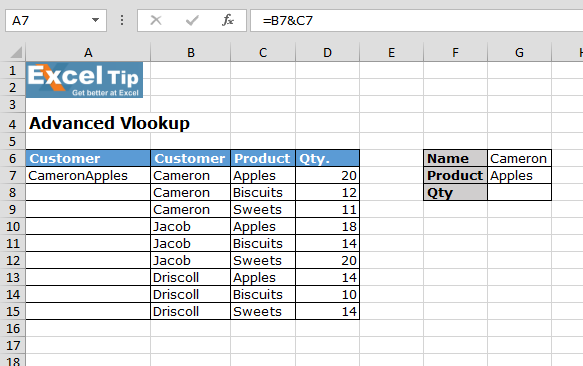• Copy the same formula in the range A8:A15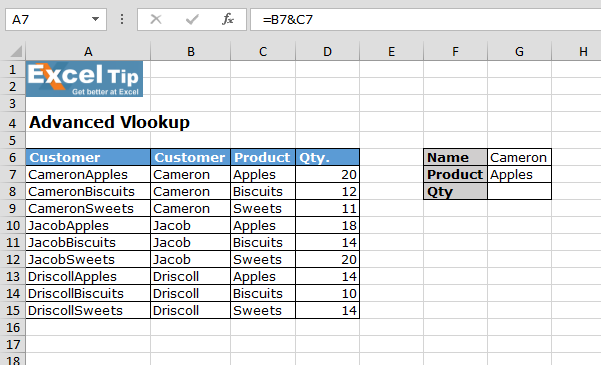Step 2:Define the range name

• Select the range A7:D15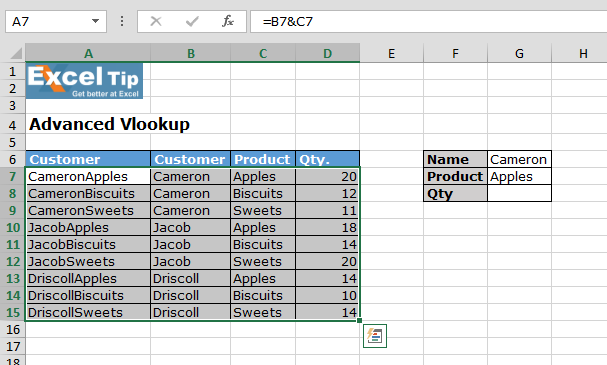• Go to Formulas tab > Defined Names group > Click on Define Name• New Name dialog box will appear
• Enter the range name
• Select the range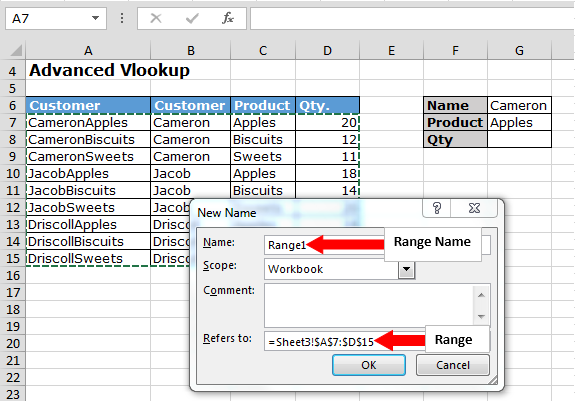• Click OK

Step 3:Enter the formula

• Enter the formula in cell G8
• =VLOOKUP(G6&G7,Range1,4,0)
• Press Enter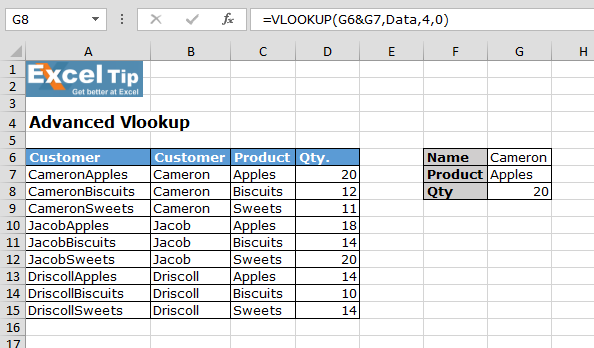• This formula will give the result according to selected options, whenever you will change the selection from the drop down list result will get change

Formula Explanation: =VLOOKUP(G6&G7,Data,4,0)

1)    =VLOOKUP(G6&G7: This part of function will help to combine the Name and product for the lookup

2)    Range1: This is our array for which we have defined the name

3)    4,0: Pickup column through lookup and 0 is define to pick the exact value1.